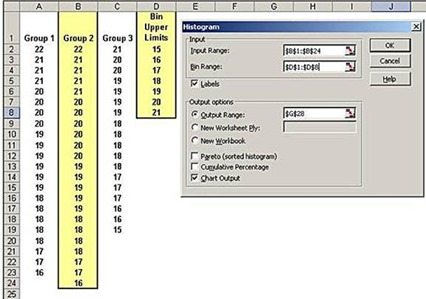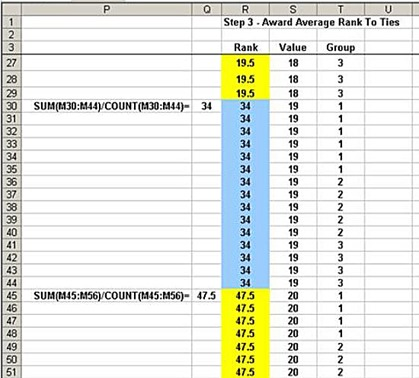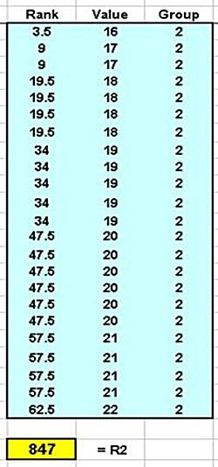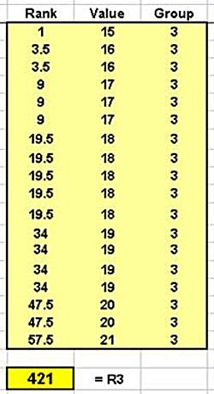## Thursday, May 29, 2014

### Kruskal-Wallis Test in 7 Steps as Nonparametric Alternative For Single-Factor ANOVA in Excel 2010 and Excel 2013

This is one of the following sixteen articles on Single-Factor ANOVA in Excel

Overview of Single-Factor ANOVA

Single-Factor ANOVA in 5 Steps in Excel 2010 and Excel 2013

Shapiro-Wilk Normality Test in Excel For Each Single-Factor ANOVA Sample Group

Kruskal-Wallis Test Alternative For Single Factor ANOVA in 7 Steps in Excel 2010 and Excel 2013

Levene’s and Brown-Forsythe Tests in Excel For Single-Factor ANOVA Sample Group Variance Comparison

Single-Factor ANOVA - All Excel Calculations

Overview of Post-Hoc Testing For Single-Factor ANOVA

Tukey-Kramer Post-Hoc Test in Excel For Single-Factor ANOVA

Games-Howell Post-Hoc Test in Excel For Single-Factor ANOVA

Overview of Effect Size For Single-Factor ANOVA

ANOVA Effect Size Calculation Eta Squared in Excel 2010 and Excel 2013

ANOVA Effect Size Calculation Psi – RMSSE – in Excel 2010 and Excel 2013

ANOVA Effect Size Calculation Omega Squared in Excel 2010 and Excel 2013

Power of Single-Factor ANOVA Test Using Free Utility G*Power

Welch’s ANOVA Test in 8 Steps in Excel Substitute For Single-Factor ANOVA When Sample Variances Are Not Similar

Brown-Forsythe F-Test in 4 Steps in Excel Substitute For Single-Factor ANOVA When Sample Variances Are Not Similar

# Kruskal-Wallis Test in 7 Steps as Nonparametric Alternative For Single- Factor ANOVA in Excel

Single-Factor ANOVA requires that the samples are taken from normally-distributed populations. If the populations are normally-distributed, the samples will be normally-distributed if the sample size is large enough, i.e., each sample contains at 15 to 20 data points.

If normality tests indicate that the samples are likely not normally-distributed, the nonparametric Kruskal-Wallis test should be substituted for Single-Factor ANOVA. The Kruskal-Wallis test is based upon the rankings of all data points and does not require that the data be normally-distributed.

The Kruskal-Wallis test does have a requirement that the data samples have similar distribution shapes. The Excel histogram is a convenient tool to quickly view the distribution shape of each sample group.

Excel histograms will be created for each sample group of the original data set. The original data set was already successfully tested for normality using the Shapiro-Wilk normality test. Excel histograms would therefore be expected to resemble the bell-shaped normal distribution curve. Histograms of each of the three data groups are shown in the following diagram:(Click On Image To See a Larger Version)

This histogram was created in Excel by inputting the following information into the histogram dialogue box:(Click On Image To See a Larger Version)(Click On Image To See a Larger Version)

This histogram was created in Excel by inputting the following information into the histogram dialogue box:(Click On Image To See a Larger Version)(Click On Image To See a Larger Version)

This histogram was created in Excel by inputting the following information into the histogram dialogue box:(Click On Image To See a Larger Version)

Excel histograms of each of the data groups reveal similar distribution shapes thus validating this required assumption of the Kruskal-Wallis test.

The Kruskall-Wallis test is based upon the overall rankings of each data point. The sum of the rankings for each sample groups, Ri, is used to calculate the value of test statistic H as follows:(Click On Image To See a Larger Version)

k = the number of sample groups

Test statistic H is very nearly distributed as the Chi-Square distribution with k – 1 degrees of freedom as long as the number of samples in each group is at least 5.

A p Value can therefore be derived from H as follows:

p value = CHISQ.DIST.RT(H, k-1)

If the p Value is smaller than the designated Level of Significance (Alpha is usually set at 0.05) then at least one of the groups has a disproportionately large share of higher numbers. A larger-than-expected share of higher numbers will produce an unexpectedly large rank sum, Ri, for the sample group. This will result in the small p Value which indicates that the difference between the rankings within sample groups is significant.

## Kruskal-Wallis Test In Excel

The Kruskal-Wallis test is performed on the original sample data as follows:(Click On Image To See a Larger Version)

### Step 1 – Arrange All Data In One Column(Click On Image To See a Larger Version)(Click On Image To See a Larger Version)(Click On Image To See a Larger Version)

### Step 2 - Sort and Then Rank the Data Column.

The data sort must keep the group number attached to each data value.(Click On Image To See a Larger Version)(Click On Image To See a Larger Version)(Click On Image To See a Larger Version)

### Step 3 – Take Care of Tied Data Values

Data whose values have ties are all assigned the same rank. This rank is the average rank that all of the same data would. This is calculation is performed as follows:(Click On Image To See a Larger Version)(Click On Image To See a Larger Version)

### Step 4 – Return Data To Original Groups

The data are then resorted back into their original group. The sort must retain the ranking for each data point.(Click On Image To See a Larger Version)(Click On Image To See a Larger Version)

### Step 5) Calculate Rank Sum For Each Group

Calculate the Rank Sum for each data group by adding the rankings of all data points in the group.(Click On Image To See a Larger Version)(Click On Image To See a Larger Version)(Click On Image To See a Larger Version)

### Using RANK.AVG() To Rank the Data

The data can be ranked and R calculated for each group much easier and quicker by using the RANK.AVG() formula as follows. Cell JF contains the following formula:

=IF(JD74=””,””,RANK.AVG(\$JD74,\$JD\$73:\$JD\$228,1))

### Step 6 – Calculate Test Statistic

Calculate test statistic based upon the following formula:(Click On Image To See a Larger Version)

Ri = Rank Sum for group i

ni = number of data points in group i

n = the total number of data points in all groups(Click On Image To See a Larger Version)

### Step 7 – Calculate the p Value

Calculate the p Value based upon h and k, the number of group as follows:(Click On Image To See a Larger Version)

The p Value formula shown here is used in Excel versions prior to 2010. The equivalent formula in Excel 2010 and later is the following:

p Value = CHISQ.DIST.RT(H, df)

This Kruskal-Wallis test does not show (just barely) a significant difference between the rankings of the sample groups. The Kruskal-Wallis test is less sensitive than Single-Factor ANOVA. This is usually the case with any nonparametric test that is used to replace a parametric test.

In this case, the Kruskal-Wallis test shows a higher chance of a type 2 error than Single-Factor ANOVA. A type 2 error is a false negative. In other words, the Kruskal-Wallis test (p value = 0.0542) is less able to detect a significant difference than Single-Factor ANOVA (p value = 0.0369), Welch’s ANOVA (p Value = 0.0463), or the Brown-Forsythe F-test (p value = 0.0378).

Welch’s ANOVA Test and the Brown-Forsythe F Tests are performed on the sample data in the 11th and 12th blog articles beyond this

Excel Master Series Blog Directory

Statistical Topics and Articles In Each Topic

• Histograms in Excel
• Bar Chart in Excel
• Combinations & Permutations in Excel
• Normal Distribution in Excel
• t-Distribution in Excel
• Binomial Distribution in Excel
• z-Tests in Excel
• t-Tests in Excel
• Hypothesis Tests of Proportion in Excel
• Chi-Square Independence Tests in Excel
• Chi-Square Goodness-Of-Fit Tests in Excel
• F Tests in Excel
• Correlation in Excel
• Pearson Correlation in Excel
• Spearman Correlation in Excel
• Confidence Intervals in Excel
• Simple Linear Regression in Excel
• Multiple Linear Regression in Excel
• Logistic Regression in Excel
• Single-Factor ANOVA in Excel
• Two-Factor ANOVA With Replication in Excel
• Two-Factor ANOVA Without Replication in Excel
• Randomized Block Design ANOVA in Excel
• Repeated-Measures ANOVA in Excel
• ANCOVA in Excel
• Normality Testing in Excel
• Nonparametric Testing in Excel
• Post Hoc Testing in Excel
• Creating Interactive Graphs of Statistical Distributions in Excel
• Solving Problems With Other Distributions in Excel
• Optimization With Excel Solver
• Chi-Square Population Variance Test in Excel
• Analyzing Data With Pivot Tables
• SEO Functions in Excel
• Time Series Analysis in Excel
• VLOOKUP